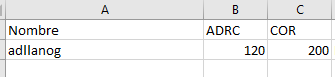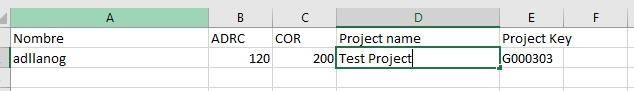# Excel source file cost person by project

Hello
My question is about importing data sources from excel files.
We would need to import cost per person data on each project. Within the Jira data source there are different projects and we would need to import an excel file in which for each person and project the cost of that person per hour is indicated and then calculate the total cost by multiplying the hourly cost in the project by the total hours allocated.
We have managed to do it per person but without linking it to a specific project.
Would there be any way to do it?

What we currently have is the following:
Excel file:Map:

We would need to map the cost per person and project with an excel similar to this one:Thank you very much in advance.

​The solution to calculate total costs requires two steps, first, additional data import with user rates for each project and second, to calculated total costs based on the spent hours and user rate in the report.

1. Hourly rate import and ​data mapping
First, select an existing data cube where you would like to import those additional data. Most likely, it is Jira data cube “Issues”.
The data source should have a column with a user name to map hourly rate to the Assignee dimension, a column with project name to map hourly rate to the Project dimension. For Assignee and Project columns also select an option Skip missing to ignore incomplete lines containing some user or project which could not be found in eazyBI data (https://docs.eazybi.com/eazybijira/data-import/jira-issues-import/additional-data-import-into-jira-issues-cube#AdditionaldataimportintoJiraIssuescube-Columnmapping)
Map columns ADRC and COR as new measures. Your data mapping screen might look like in the picture below:

​2. Calculated measure in the report
After data import, you will have new user defined measures “ADRC” and “COR”. To get the total costs for each assignee or project, define a new calculated measure in Measures and multiply Original estimated hours with “ADRC” (or “COR”). See picture below:

You may analyze Total costs by Project and Assignee dimensions (dimensions which are in data mapping screen from step 1.)

Best,
Zane / support@eazyBI.com